$\underbrace{\left[\begin{array}{cc} 1 & 1 \\ 0 & 1 \end{array}\right]}_{\text {Shear }}(\underbrace{\left[\begin{array}{cc} 0 & -1 \\ 1 & 0 \end{array}\right]}_{\text {Rotation }}\left[\begin{array}{l} x \\ y \end{array}\right])=\underbrace{\left[\begin{array}{cc} 1 & -1 \\ 1 & 0 \end{array}\right]}_{\text {Composition }} \left[\begin{array}{l} x \\ y \end{array}\right]$

## 行列式

$\operatorname{det}\left(\left[\begin{array}{ll} a & b \\ c & d \end{array}\right]\right)=a d-b c$

## 线性方程组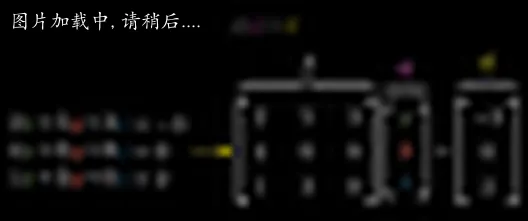## 叉乘 Cross product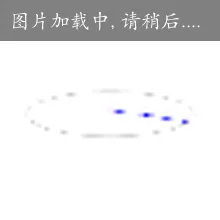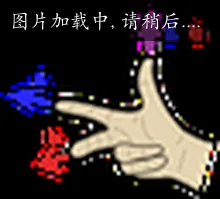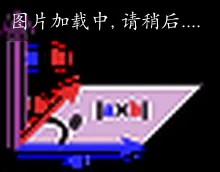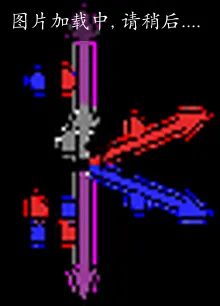## 编程与线性代数

Complexity will go somewhere: if not the language then the application code.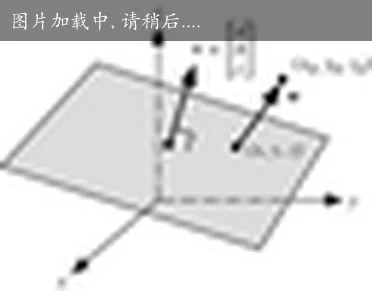## 参考

If you have any questions or feedback, please reach out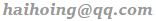.